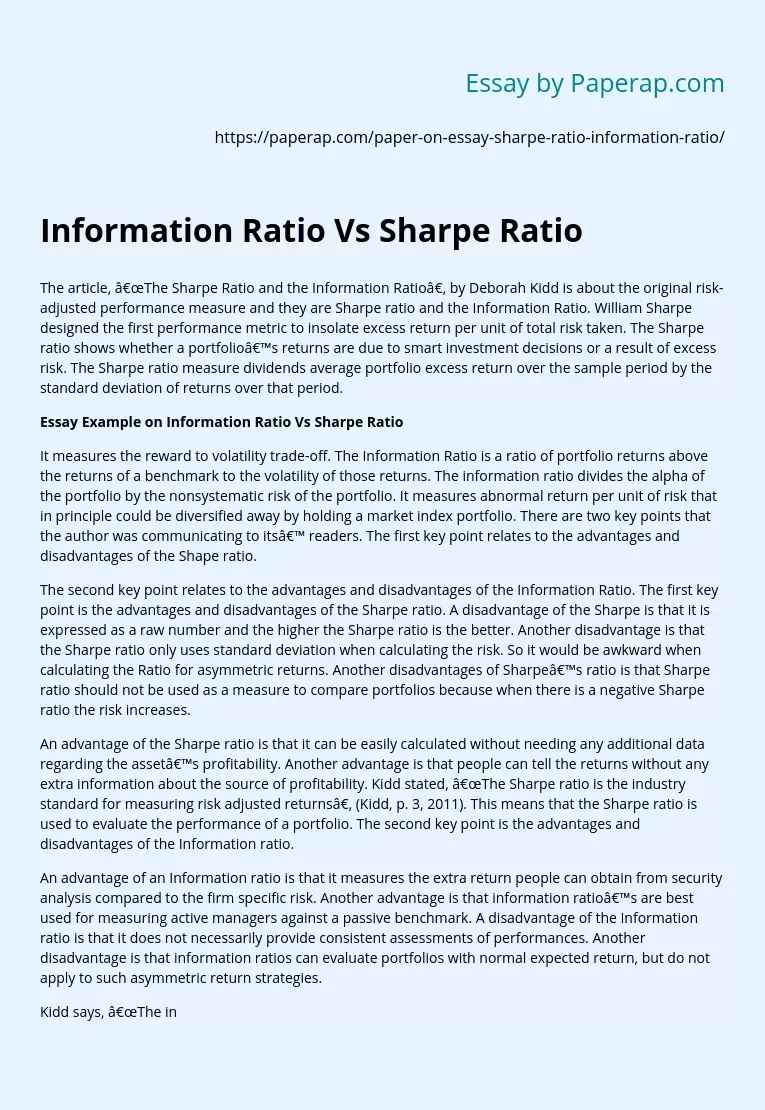# Information Ratio Vs Sharpe Ratio

Topics: Economics

The article, “The Sharpe Ratio and the Information Ratio”, by Deborah Kidd is about the original risk-adjusted performance measure and they are Sharpe ratio and the Information Ratio. William Sharpe designed the first performance metric to insolate excess return per unit of total risk taken. The Sharpe ratio shows whether a portfolio’s returns are due to smart investment decisions or a result of excess risk. The Sharpe ratio measure dividends average portfolio excess return over the sample period by the standard deviation of returns over that period.

## Essay Example on Information Ratio Vs Sharpe Ratio

It measures the reward to volatility trade-off. The Information Ratio is a ratio of portfolio returns above the returns of a benchmark to the volatility of those returns. The information ratio divides the alpha of the portfolio by the nonsystematic risk of the portfolio. It measures abnormal return per unit of risk that in principle could be diversified away by holding a market index portfolio.

There are two key points that the author was communicating to its’ readers. The first key point relates to the advantages and disadvantages of the Shape ratio.

The second key point relates to the advantages and disadvantages of the Information Ratio. The first key point is the advantages and disadvantages of the Sharpe ratio. A disadvantage of the Sharpe is that it is expressed as a raw number and the higher the Sharpe ratio is the better. Another disadvantage is that the Sharpe ratio only uses standard deviation when calculating the risk.

Get quality help nowWriterBelleVerified

Proficient in: Economics4.7 (657)

“ Really polite, and a great writer! Task done as described and better, responded to all my questions promptly too! ”+84 relevant experts are online

So it would be awkward when calculating the Ratio for asymmetric returns. Another disadvantages of Sharpe’s ratio is that Sharpe ratio should not be used as a measure to compare portfolios because when there is a negative Sharpe ratio the risk increases.

An advantage of the Sharpe ratio is that it can be easily calculated without needing any additional data regarding the asset’s profitability. Another advantage is that people can tell the returns without any extra information about the source of profitability. Kidd stated, “The Sharpe ratio is the industry standard for measuring risk adjusted returns”, (Kidd, p. 3, 2011). This means that the Sharpe ratio is used to evaluate the performance of a portfolio. The second key point is the advantages and disadvantages of the Information ratio.

An advantage of an Information ratio is that it measures the extra return people can obtain from security analysis compared to the firm specific risk. Another advantage is that information ratio’s are best used for measuring active managers against a passive benchmark. A disadvantage of the Information ratio is that it does not necessarily provide consistent assessments of performances. Another disadvantage is that information ratios can evaluate portfolios with normal expected return, but do not apply to such asymmetric return strategies.

Kidd says, “The information ratios is often referred to as a variation or generalized version of the Sharpe ratio” (Kidd, p. 2, 2011), which means they are similar, but the Information ratios branches off. That is because information ratio has been doing better than stocks on a risk-adjusted basis on higher return. When used correctly, the Sharpe ratio and the information ratio can be valuable tools. These ratios are most useful for evaluating portfolios with normal expected return distributions, (Kidd, 2011). However, these ratios could potentially be misleading if used naively.

Both the Sharpe ratio and the Information ratio, does not take into account dynamic correlations between asset classes. As it relates to investors, it is important for them to be mindful that long periods may increase a portfolio’s Sharpe ratio due to lower volatility for long periods. As far as the information ratios, they are based on longer periods, which results for them being more valuable because they are persistent in manager skill.

References Kidd, D. (2011). The sharpe ratio and the information ratio. Investment Performance Measurement, 1-3.